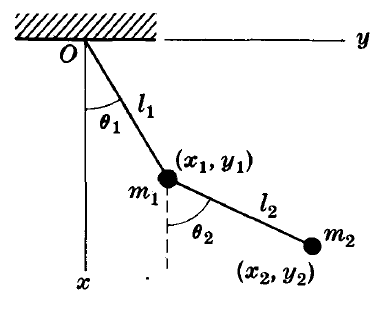# Scleronomic or Rheonomic Mechanical System?

Gold Member
I would really appreciate if someone could advise me whether the system below is a scleronomic or a rheonomic mechanical system, or a mix of both. If we consider the first pendulum, the constraint is fixed which leads to a scleronomous case while the constraint of the second pendulum is not fixed (varies with time) which leads to a rheonomous case.#### Attachments

berkeman
Mentor
Is this a schoolwork question?

wrobel
Present equations of constraints as ##f_i(x_1,y_1,x_2,y_2)=0,\quad i=1,2##

Gold Member
Is this a schoolwork question?
No

•berkeman
No, the constraints here are not the pendulums but the rod that connects the pendulum. Now, try to find the equations that describe each constraint (i.e. the constraint equations) in the inertial frame of reference. Put all terms on the LHS (i.e. set RHS equals 0). This is then called the Pffafian form of the constraint. The definition of a scleronomic system is that the constraint equations of the system relate only the positions of the masses in the system, can be arranged into the Pffafian form. Since you can find a Pffafian form of the constraints, you have a scleronomic system. An example of a rheonomic system would be a rolling disk in 3 dimensions, in which the constraint equation relates the positions as well as velocities of the masses of the system, and in particular it cannot be integrated to a constraint equation that only relates the positions of the masses in the system.

wrobel